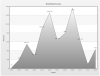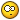## Recommended Posts

Hello -

I hope that I am in the correct forum for this.

I am trying to create an area chart. Do you know how I specify the numbers that display on the left side? I need to have them as 0, 200, 400, 600, 800, 1000, 1200, 1400. I do not want any symbols such as \$, %, etc.

I should also add that the bottom X axis labels go from 2001 - 2012. Then there are many data points (in decimal format) in the XML file. Data points include years 2001-2012.

Many thanks!

Edited by dcwebcat

##### Share on other sitesHi,

1. To remove symbols such as '\$', '%' etc. please set the "numberPrefix" and "numberSuffix" attribute values to NULL in the <chart> element of your XML data.

2. To display Y-Axis labels as 0, 200, 400, 600, 800, 1000, 1200, 1400, you need to set the following attributes in the <chart> element of your XML data.

```<chart formatNumber='0' formatNumberScale='0' adjustDiv='0' yAxisMaxvalue='1400' yAxisMinValue='0' numDivLines='6'>
```

3. To display the X-Axis labels from 2001 - 2012, you need to set the years for each data points as mentioned below:

```<set label='2001' value='20' ... />
<set label='2002' value='200' ... />
....
<set label='2012' value='432' />
```

Please find the attached screen shot of the same for your reference.##### Share on other sites

Thank you. This is exactly what I need to accomplish but I am having a hard time getting it to work.

Below is my HTML code and the XML code. Is there anything obvious that I am doing wrong? Any help is greatly appreciated!!!XML CODE:

<chart bgColor='E9E9E9' outCnvBaseFontColor='666666' caption='' xAxisName='' yAxisName='' numberPrefix='' showLabels='1' formatNumber='0' formatNumberScale='0' adjustDiv='0' yAxisMaxvalue='1400' yAxisMinValue='0' numDivLines='6' showValues='0' plotFillAlpha='70' showAlternateVGridColor='1' AlternateVGridColor='e1f5ff' divLineColor='e1f5ff' vdivLineColor='e1f5ff' baseFontColor='666666' canvasBorderThickness='1' showPlotBorder='1' plotBorderThickness='0' canvaspadding='0' >

<categories>

<category label='2001' />

<category label='2002' />

<category label='2003' />

<category label='2004' />

<category label='2005' />

<category label='2006' />

<category label='2007' />

<category label='2008' />

<category label='2009' />

<category label='2010' />

<category label='2011' />

<category label='2012' />

</categories>

<dataset seriesName='' color='B1D1DC' plotBorderColor='B1D1DC'>

<set label='2001' value='1.54' />

<set label='2001' value='1.36' />

<set label='2001' value='1.38' />

<set label='2001' value='1.06' />

<set label='2002' value='1.36' />

<set label='2003' value='1.07' />

<set label='2004' value='0.88' />

<set label='2005' value='0.69' />

<set label='2006' value='0.76' />

<set label='2007' value='0.62' />

<set label='2008' value='0.47' />

<set label='2009' value='1.07' />

<set label='2010' value='0.65' />

<set label='2011' value='1.23' />

<set label='2012' value='2.24' />

</dataset>

</chart>

HTML CODE:

<html>

<title>My First chart using FusionCharts</title>

<script type="text/javascript" src="FusionCharts/FusionCharts.js">

</script>

<body>

<script type="text/javascript"><!--

var myChart = new FusionCharts( "FusionCharts/Area2D.swf",

"myChartId", "785", "600", "0", "1" );

myChart.setXMLUrl("chart1.xml");

myChart.render("chartContainer");

// -->

</script>

</body>

</html>

##### Share on other sites

Well, I updated the XML file and now the chart looks good as far as the X and Y axis labels displaying but the data is not showing up. It's just blank.<chart bgColor='E9E9E9' outCnvBaseFontColor='666666' caption='' xAxisName='' yAxisName='' numberPrefix='' showLabels='1' formatNumber='0' forceDecimals='1' formatNumberScale='0' adjustDiv='0' yAxisMaxvalue='1400' yAxisMinValue='0' numDivLines='6' showValues='0' plotFillAlpha='70' showAlternateVGridColor='1' AlternateVGridColor='e1f5ff' divLineColor='e1f5ff' vdivLineColor='e1f5ff' baseFontColor='666666' canvasBorderThickness='1' showPlotBorder='1' plotBorderThickness='0' canvaspadding='0' >

<set label='2001' value='1.54' />

<set label='2001' value='1.36' />

<set label='2001' value='1.38' />

<set label='2001' value='1.06' />

<set label='2002' value='1.36' />

<set label='2003' value='1.07' />

<set label='2004' value='0.88' />

<set label='2005' value='0.69' />

<set label='2006' value='0.76' />

<set label='2007' value='0.62' />

<set label='2008' value='0.47' />

<set label='2009' value='1.07' />

<set label='2010' value='0.65' />

<set label='2011' value='1.23' />

<set label='2012' value='2.24' />

</chart>

##### Share on other sites

Hey,

yAxisMaxValue attribute has been defined with a higher value when compared to the data plot values. So, the data plot values are small when compared to defined Y-axis maximum value.

Remove this attribute and a good looking chart will be rendered.